Next: Linear systems Up: Theory Previous: Theory   Contents

## Dynamical systems

The theory of dynamical systems is the basic mathematical tool for analysing time series. This section presents a brief introduction to the basic concepts. For a more extensive treatment, see for example .

The general form for an autonomous discrete-time dynamical system is the map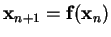(2.1)

where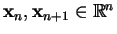and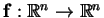is a diffeomorphism, i.e. a smooth mapping with a smooth inverse. It is important that the mapping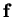is independent of time, meaning that it only depends on the argument point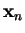. Such mappings are often generated by flows of autonomous differential equations.

For a general autonomous differential equation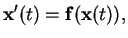(2.2)

we define the flow by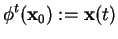(2.3)

where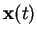is the unique solution of Equation (2.2) with the initial condition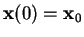, evaluated at time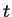. The functionin Equation (2.2) is called the vector field corresponding to the flow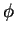.

Setting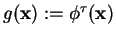, where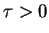, gives an autonomous discrete-time dynamical system like in Equation (2.1). The discrete system defined in this way samples the values of the continuous system at constant intervals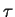. Thus it is a discretisation of the continuous system.Next: Linear systems Up: Theory Previous: Theory   Contents
Antti Honkela 2001-05-30